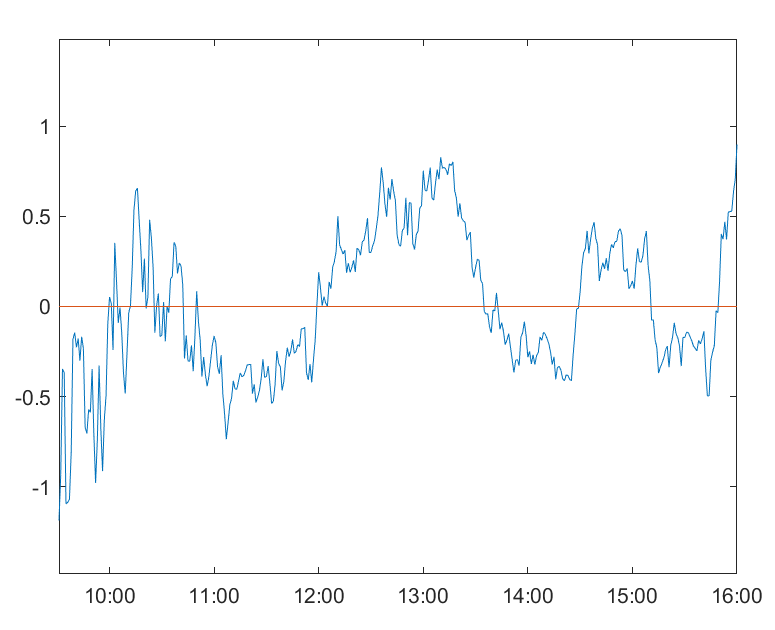### 課程大綱

[注意：本課程以理論為主軸]

1. 認識金融商品與金融交易

• 實質資產 vs. 金融資產

• 債券、權益證券、衍生性金融商品

• 配對交易概念

2. 除權息、還原價與績效設定 (不實作)

• 除權息與參考價

• 還原價與客製化還原設計

• 常見報酬率參考值

1. 算術平均 (arithmetic mean)

2. 幾何平均 (geometric mean)

3. 內在報酬率 (internal rate of return, IRR)

4. 年利率 (annual percentage rates, APR)

5. 有效年利率 (effective annual rate, EAR)

6. 持有期間報酬率 (holding-period return rate, HPR)

7. 總報酬率 (total return rate, TRR)

• 持有期間報酬及總報酬對於交易策略數量之關聯

• 常見績效指標

1. 夏普比率 (Sharpe ratio)

2. 標準報酬 (standard return)

3. M 平方值 (M-square)

4. 崔納衡量值 (Treynor measure)

5. 資訊比率 (information ratio)

3. 理論與模型建立及分析

• 套利定價理論 (arbitrage pricing theory, APT)

• 現金流與投資組合價值之差異

• 線性方程組 (linear system) 與線性迴歸 (linear regression)

1. 普通線性迴歸 (ordinary linear regression)

2. 多變量線性迴歸 (multivariate linear regression)

3. 多變量化約秩迴歸 (multivariate reduced-rank regression, multivariate RRR)

4. 向量自我迴歸 (vector auto-regression, VAR)

5. 向量誤差修正模型 (vector error-correction model, VECM)

• 常見估計量

1. 普通最小平方法估計量 (ordinary least-squares estimator, OLS estimator)

2. 最大概似估計量 (maximum likelihood estimator, MLE)

3. 受限最小平方估計量 (restricted least-squares estimator, RLS estimator)

4. 主成份估計量 (principal components estimator, PCE)

5. 共整合估計量 (cointegration estimator)

• 常見檢定

1. 受限最小平方檢定 (restricted least-squares test)

2. 線性檢定 (linearity test) 之潤瑟迴歸設定檢定 (Ramsey RESET)

3. 常態檢定 (normality test) 之哈爾克--貝拉檢定 (Jarque--Bera test, JB test)

4. 共整合檢定 (cointegration test) 之 Johansen 檢定 (Johansen test)

• 配對組合適用性測量與隨機搜索投資組合

• 均值回復技術指標與趨勢技術指標

• 本課程以中文教材為主，但仍有部分文字語言為英文，同時教材僅部分使用。

• 本課程預計會安排程式考試一次。

• 實際授課內容需視課堂學員學習情況而定。

• 為配合當下最新 Python 版本，本課程不建議學員使用虛擬環境。

• 本課程會提供雲端硬碟供學員下載上課的程式碼，於課程結束後一週關閉。

• 本課程預計會安排之學習評量，可於課程網站中參考上期試卷題目。

• 課程網站請參考 https://www.csie.ntu.edu.tw/~d06922002/Course/PTS/

### 適合對象

• 嫻熟於使用 Python 者

• 證券或相關金融商品交易經驗者

• 對線性代數與機率論基礎有所了解者

### 開發環境

• Python 3.8.5

• NumPy 1.19.1

• SciPy 1.5.2

• matplotlib 3.3.0

• influxdb 5.3.0

### 注意事項

• 上課地點：台灣大學資訊工程學系系館　詳細位置；教室號碼將於開課前一日公佈於官網"最新消息"中。
• 上課教材：開課後將公布教學網站給同學查閱。
• 結業狀況：請上官網”結業名單”查閱是否合格及可領取證書進度。

### 講師介紹

(一) 學歷：

• 國立交通大學應用數學所碩士學位
• 國立台灣大學資訊工程學系博士班

(二) 經歷：

(三) 專長：

• 統計套利 (statistical arbitrage)